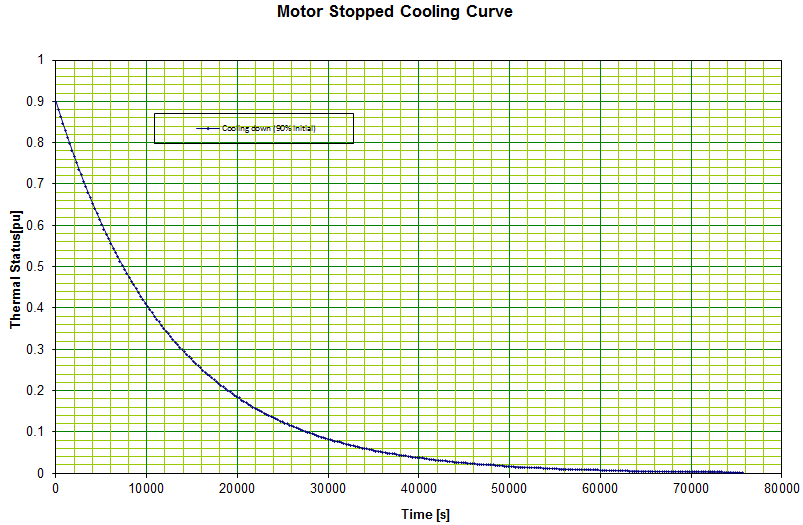###### Cooling time of an Induction motorCooling status is referred to the condition defined by a motor temperature reduction caused by a decreasing current from running condition to a less than the Full Load Amps (FLA)​​ condition known as minimum running condition. The cooling status is also initiated by a stopped motor following a running condition.

Motor thermal status, also referred as motor thermal capacity is a parameter used to evaluate the thermal condition of the​​ motor.

Thermal status is measured in percentage of the motor thermal limit. When a motor is stopped for a long time, the thermal status is 0% which means that zero percent of its capacity is used. At motor starting, the thermal capacity used can reach let's said 40%. If the motor has two cold starts, then the thermal capacity after these actions would be 80%. Exceeding the thermal capacity of the motor produces a loss of insulation life due to deterioration by overheating the windings.​​

During the cooling time, the thermal status decreases from a higher running thermal status to a lower one.

The equation of the cooling motor is given by:

θactual=θfinal+θinitial-θfinale-t/τ

where:

θinitial:​​ Thermal status at the start of the cooling period (t= 0) in percent

θfinal   :​​ Thermal status at the end of the cooling period (t → ꝏ) in percent

θactual:​​ Thermal status after time​​ t​​ in percent

t           :​​ Time in seconds

τ           :​​ Cooling time constant in seconds

Thermal status is proportional to I squared and the equation can be expressed in the following form:​​

I2(t)=I2f+I20-I2fe-t/τ

where:

I20:​​ Motor current squared at the start of the cooling period (t= 0) in per unit (pu)

I2f :​​ Motor current squared at the end of the cooling period (t → ꝏ) in pu

I2(t):​​ Motor current squared after time​​ t​​ in pu

t      :​​ Time in seconds

τ      :​​ Cooling time constant in seconds

Example

A motor with a cooling time​​ constant of 3.5 hours is stopped with a thermal status of 90%. Calculate the time required to reach 40% motor status.

Replacing the data in the first equation gives:

40=0+90-0e-t/12600

t=-12600 ln4090=10217 s

The required time is 2 hours 50 minutes.

The curve below shows the motor thermal status​​ variation with time for this example.​​Ernesto Hidalgo-Tupia, Eng., M. A. Sc.​​
Electrical EngineerDownload PDF